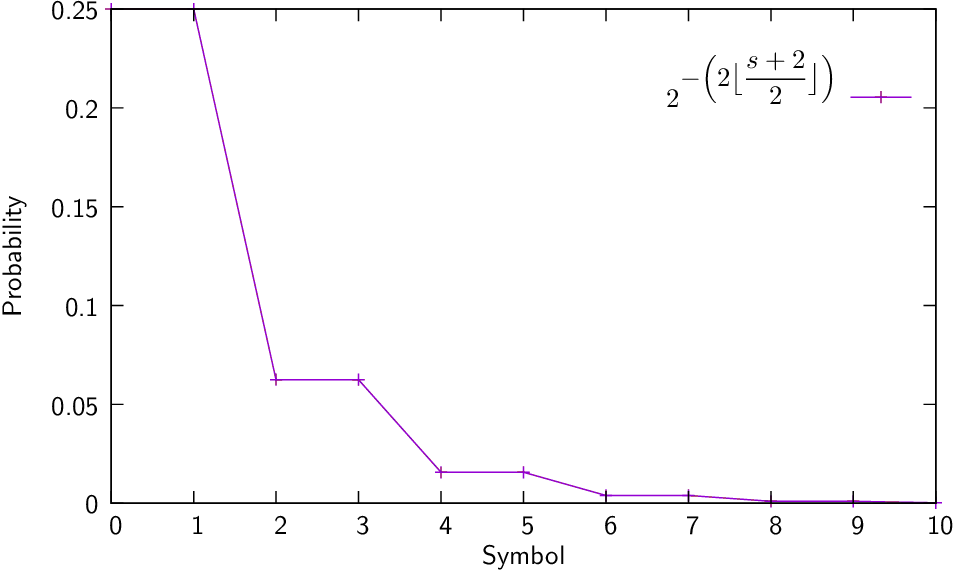## Golomb and Rice coding

July 10, 2017

### 1 Fundamentals

• S.W. Golomb designs in 1966 an alternative to the Huﬀman coding .
• When the probabilities of the symbols follow an exponential distribution, the Golomg encoder has the same eﬃciency than the Huﬀman coding, but it is faster. In this case, the probabilities of the symbols shoud be  $p\left(s\right)={2}^{-\left(\rightm⌊\frac{s+m}{m}⌋\left)\right}$ (Eq:Golomb)

where $s=0,1,\cdots \phantom{\rule{0.3em}{0ex}}$ is the symbol and $m=0,1,\cdots \phantom{\rule{0.3em}{0ex}}$ is the “Golomb slope” of the distribution.

• For $m={2}^{k}$, it is possible to ﬁnd a very eﬃcient implementation and the encoder is also named Rice encoder . In this case  $p\left(s\right)={2}^{-\left(\right{2}^{k}⌊\frac{s+{2}^{k}}{{2}^{k}}⌋\left)\right}$ (Eq:Rice)

 Golomb $m=1$ $m=2$ $m=3$ $m=4$ $m=5$ $m=6$ $m=7$ $m=8$ Rice $k=0$ $k=1$ $k=2$ $k=3$ $s=0$ 0 00 00 000 000 000 000 0000 1 10 01 010 001 001 001 0010 0001 2 110 100 011 010 010 0100 0011 0010 3 1110 101 100 011 0110 0101 0100 0011 4 11110 1100 1010 1000 0111 0110 0101 0100 5 ${1}^{5}0$ 1101 1011 1001 1000 0111 0110 0101 6 ${1}^{6}0$ 11100 1100 1010 1001 1000 0111 0110 7 ${1}^{7}0$ 11101 11010 1011 1010 1001 1000 0111 8 ${1}^{8}0$ 111100 11011 11000 10110 10100 10010 10000 $⋮$ $⋮$ $⋮$ $⋮$ $⋮$ $⋮$ $⋮$ $⋮$ $⋮$
• Notice that for $m=1$, we take the unary encoding.### 2 The Golomb encoder

1. $k←⌈{log}_{2}\left(m\right)⌉$.
2. $r←s\phantom{\rule{1em}{0ex}}\mathrm{mod}\phantom{\rule{1em}{0ex}}m$.
3. $t←{2}^{k}-m$.
4. Output $\left(s\phantom{\rule{1em}{0ex}}\mathrm{div}\phantom{\rule{1em}{0ex}}m\right)$ using an unary code.
5. If $r:
1. Output the integer encoded in the $k-1$ least signiﬁcant bits of $r$ using a binary code.
6. Else:
1. $r←r+t$.
2. Output the integer encoded in the $k$ least signiﬁcant bits of $r$ using a binary code.

### Example ($m=7$ and $s=8$)

1. $k←⌈{log}_{2}\left(8\right)⌉=3$.
2. $r←8\text{mod}7=1$.
3. $t←{2}^{3}-7=8-7=1$.
4. Output $←8\text{div}7=⌊8∕7⌋=1$ as an unary code (2 bits).
Therefore, output $←10$.
5. $r=1\le t=1$.
6. $r←1+1=2$.
7. Output $r=2$ using a binary code of $k=3$ bits.
Therefore, $c\left(8\right)=10010$.

### 3 The Golomb decoder

1. $k←⌈{log}_{2}\left(m\right)⌉$.
2. $t←{2}^{k}-m$.
3. Let $s←$ the number of consecutive ones in the input (we stop when we read a $0$).
4. Let $x←$ the next $k-1$ bits in the input.
5. If $x:
1. $s←s×m+x$.
6. Else:
1. $x←x×2\phantom{\rule{1em}{0ex}}+$ next input bit.
2. $s←s×m+x-t$.

### Example (decode $10010$ where $m=7$)

1. $k←3$.
2. $t←{2}^{k}-m={2}^{3}-7=1$).
3. $s←1$ because we found only one $1$ in the input.
4. $x←{\text{input}}_{k-1}={\text{input}}_{2}=01$.
5. $x=1\nless t=1$.
6. .
7. $s←s×m+x-t=1×7+2-1=8$.

### Let’s go the the lab!

1. make golomb.
2.                     Codec | lena boats pepers zelda Average
--------------------------+--------------------------------
: |    :     :      :     :       :
golomb | ....  ....   ....  ....    ....
tpt 1 | golomb | ....  ....   ....  ....    ....

Note: this implementation of the Golomb encoding estimates the $m$ parameter automatically.

### 4 The Rice encoder

1. $m←{2}^{k}$.
2. Output $←⌊s∕m⌋$ using an unary code ($⌊s∕m⌋+1$ bits).
3. Output $←$ the $k$ least signiﬁcant bits of $s$ using a binary code.

### Example ($k=1$ and $s=7$)

1. $m←{2}^{k}={2}^{1}=2$.
2. Output $←⌊s∕m⌋=⌊7∕2⌋=3$ using an unary code of 4 bits. Therefore, output $←1110$.
3. Output $←$ the $k=1$ least signiﬁcant bits of $s=7$ using a unary code ($k+1$ bits). So, output $←1$. Total output $c\left(7\right)=11101$.

### 5 The Rice decoder

1. Let $s$ the number of consecutive ones in the input (we stop when we read a 0).
2. Let $x$ the next $k$ input bits.
3. $s←s×{2}^{k}+x$.

### Example (decode $11101$ where $k=1$)

1. $s←3$ because we found $3$ consecutive ones in the input.
2. $x←$ next input $k=1$ input bits. Therefore $x←1$.
3. $s←s×{2}^{k}+x=3×{2}^{1}+1=6+1=7$.

### Let’s go the the lab!

1. make rice.
2.                     Codec | lena boats pepers zelda Average
--------------------------+--------------------------------
: |    :     :      :     :       :
rice | ....  ....   ....  ....    ....
tpt 1 | rice | ....  ....   ....  ....    ....

Note: this implementation of the Rice encoding estimates the $k$ parameter automatically.

### References

   S.W. Golomb. Run-Length Encodings. IEEE Transactions on Information Theory, 12:399–401, 1966.

   R. F. Rice. Some Practical Universal Noiseless Coding Techniques. Technical Report 79/22, Jet Propulsion Laboratory, 1979.